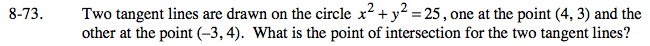### Home > PC > Chapter 8 > Lesson 8.2.1 > Problem8-73

8-73.Find the equations of the tangents by using the point-slope form.
The slopes of the tangents are the reciprocals of the slopes of the corresponding radii multiplied by −1.

Set the two equations equal to each other and solve for the coordinates of the intersection.

(1,7)

Use the eTool below to help solve this problem by graphing.
Click the link at right for the full version of the eTool: PCT 8-73 HW eTool